# 09-240/Classnotes for Tuesday September 22

## Contents

WARNING: The notes below, written for students and by students, are provided "as is", with absolutely no warranty. They can not be assumed to be complete, correct, reliable or relevant. If you don't like them, don't read them. It is a bad idea to stop taking your own notes thinking that these notes can be a total replacement - there's nothing like one's own handwriting! Visit this pages' history tab to see who added what and when.

## Class notes for today

Vectors:

2. can be multiplied by a number (not another vector)

Let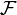$\mathcal F$ be a field. A vector space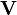$\mathbf V$ over the field$\mathcal F$ is a set$\mathbf V$ (of vectors) with a special element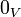$0_V$, a binary operation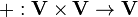$+ : \mathbf V \times \mathbf V \rightarrow \mathbf V$, a binary operation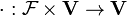$\cdot : \mathcal F \times \mathbf V \rightarrow \mathbf V$.

 Convention for today: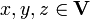$x, y, z \in \mathbf V$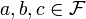$a, b, c \in \mathcal F$

VS1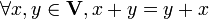$\forall x, y \in \mathbf V, x + y = y + x$
VS2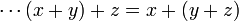$\cdots (x + y) + z = x + (y + z)$
VS3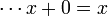$\cdots x + 0 = x$
VS4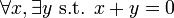$\forall x, \exists y \mbox{ s.t. } x + y = 0$
VS5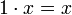$1 \cdot x = x$
VS6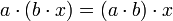$a \cdot (b \cdot x) = (a \cdot b) \cdot x$
VS7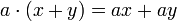$a \cdot (x + y) = ax + ay$
VS8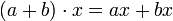$(a + b) \cdot x = ax + bx$

### Proof of VS4

Take an arbitrary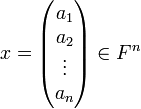$x = \begin{pmatrix} a_1 \\ a_2 \\ \vdots \\ a_n \end{pmatrix} \in F^n$

Set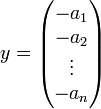$y = \begin{pmatrix} -a_1 \\ -a_2 \\ \vdots \\ -a_n \end{pmatrix}$ and note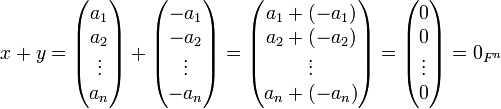$x + y = \begin{pmatrix} a_1 \\ a_2 \\ \vdots \\ a_n \end{pmatrix} + \begin{pmatrix} -a_1 \\ -a_2 \\ \vdots \\ -a_n \end{pmatrix} = \begin{pmatrix} a_1 + (-a_1) \\ a_2 + (-a_2) \\ \vdots \\ a_n + (-a_n) \end{pmatrix} = \begin{pmatrix} 0 \\ 0 \\ \vdots \\ 0 \end{pmatrix} = 0_{F^n}$

### Examples

1.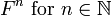$F^n \mbox{ for } n \in \mathbb N$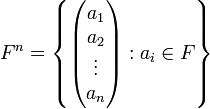$F^n = \left\{ \begin{pmatrix} a_1 \\ a_2 \\ \vdots \\ a_n \end{pmatrix} : a_i \in F \right\}$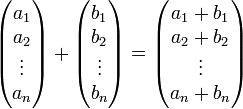$\begin{pmatrix} a_1 \\ a_2 \\ \vdots \\ a_n \end{pmatrix} + \begin{pmatrix} b_1 \\ b_2 \\ \vdots \\ b_n \end{pmatrix} = \begin{pmatrix} a_1 + b_1 \\ a_2 + b_2 \\ \vdots \\ a_n + b_n \end{pmatrix}$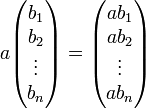$a \begin{pmatrix} b_1 \\ b_2 \\ \vdots \\ b_n \end{pmatrix} = \begin{pmatrix} ab_1 \\ ab_2 \\ \vdots \\ ab_n \end{pmatrix}$
...
2.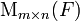$\mathrm M_{m \times n}(F)$
...
3.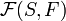$\mathcal F(S, F)$
4. Polynomials
5.$...$

### Food for thought

What is wrong with setting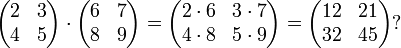$\begin{pmatrix} 2 & 3 \\ 4 & 5 \\ \end{pmatrix} \cdot \begin{pmatrix} 6 & 7 \\ 8 & 9 \\ \end{pmatrix} = \begin{pmatrix} 2 \cdot 6 & 3 \cdot 7 \\ 4 \cdot 8 & 5 \cdot 9 \\ \end{pmatrix} = \begin{pmatrix} 12 & 21 \\ 32 & 45 \\ \end{pmatrix} ?$

1. Unnecessary for a V.S.
2. This is useless, since it does not describe reality. For example, a mathematical theory with 46 dimensions can be perfect and mathematically elegant, but if the only solution to it is a universe in which life cannot form it is not reality, hence we have no use for it.